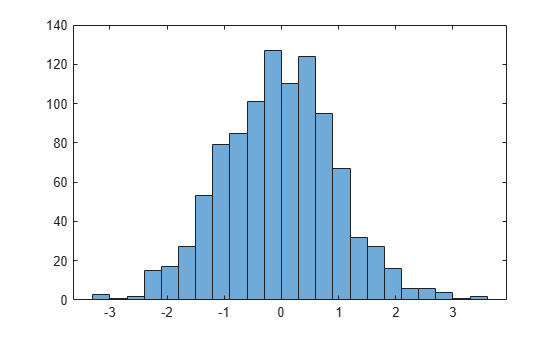# morebins

Increase number of histogram bins

## Syntax

``N = morebins(h)``
``N = morebins(h,direction)``

## Description

example

````N = morebins(h)` increases the number of bins in histogram `h` by 10% (rounded up to the nearest integer) and returns the new number of bins.For bivariate histograms, this increases the bin count in both the x and y directions.```
````N = morebins(h,direction)`, where `h` must be a `histogram2` object, only increases the number of bins in the dimension specified by `direction`. The `direction` option can be `'x'`, `'y'`, or `'both'`. The default value is `'both'`.```

## Examples

collapse all

Plot a histogram of 1,000 random numbers and return a handle to the histogram object.

```x = randn(1000,1); h = histogram(x)``````h = Histogram with properties: Data: [1000x1 double] Values: [3 1 2 15 17 27 53 79 85 101 127 110 124 95 67 32 27 16 6 6 4 1 2] NumBins: 23 BinEdges: [-3.3000 -3.0000 -2.7000 -2.4000 -2.1000 -1.8000 -1.5000 -1.2000 -0.9000 -0.6000 -0.3000 0 0.3000 0.6000 0.9000 1.2000 1.5000 1.8000 2.1000 2.4000 2.7000 3 3.3000 3.6000] BinWidth: 0.3000 BinLimits: [-3.3000 3.6000] Normalization: 'count' FaceColor: 'auto' EdgeColor: [0 0 0] Show all properties ```

Use `morebins` to increase the number of bins in the histogram.

```morebins(h); morebins(h)``````ans = 29 ```

## Input Arguments

collapse all

Input histogram, specified as a `histogram` or `histogram2` object.

`h` cannot be a categorical histogram.

Direction to increase the number of bins, specified as `'x'`, `'y'`, or `'both'`. Specify `'x'` or `'y'` to only increase the number of bins in that direction while leaving the number of bins in the other direction constant.

## Output Arguments

collapse all

Number of bins, returned as a scalar or vector. `N` is the new number of bins for the histogram after increase. For bivariate histogram plots, `N` is a two-element vector, ```[nx ny]```.

## Version History

Introduced in R2014b